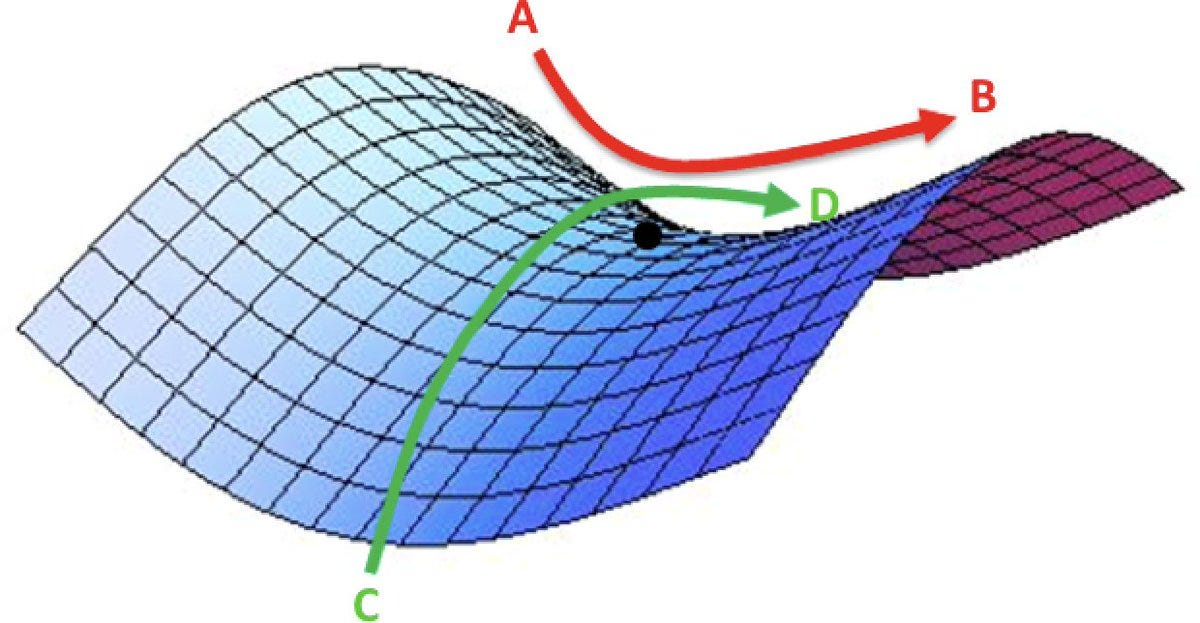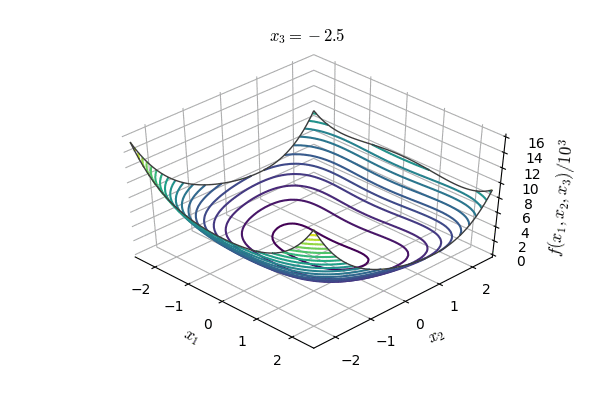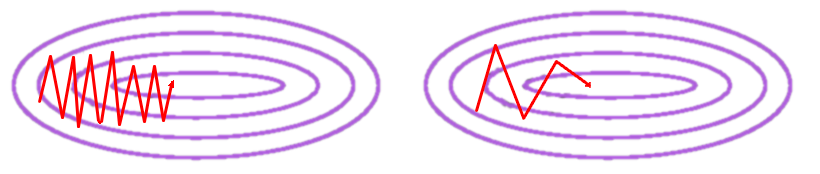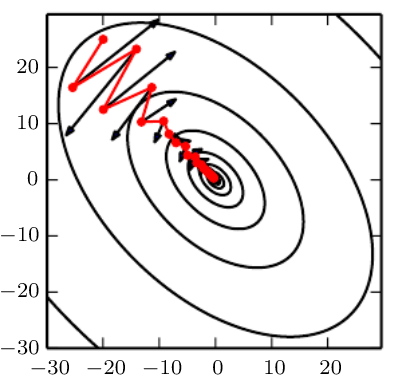# Neural Network Optimization

Deep LearningModelingNeural Networksposted by Matthew Stewart April 15, 2020

This article is the third in a series of articles aimed at demystifying neural networks and outlining how to design and...• Challenges with optimization
• Momentum
• Parameter Initialization
• Batch Normalization
These tutorials are largely based on the notes and examples from multiple classes taught at Harvard and Stanford in the computer science and data science departments.

[Related Article: Image Augmentation for Convolutional Neural Networks]

# Challenges with Optimization

When talking about optimization in the context of neural networks, we are discussing non-convex optimization.

• How do we avoid getting stuck in local optima? One local optimum may be surrounded by a particularly steep loss function, and it may be difficult to ‘escape’ this local optimum.
• What if the loss surface morphology changes? Even if we can find the global minimum, there is no guarantee that it will remain the global minimum indefinitely. A good example of this is when training on a dataset that is not representative of the actual data distribution—when applied to new data, the loss surface will different. This is one reason why trying to make the training and test datasets representative of the total data distribution is of such high importance. Another good example is data that habitually changes in distribution due to its dynamic nature—an example of this would be user preferences for popular music or movies, which changes day-to-day and month-to-month.

## Local Optima

Previously, local minima were viewed as a major problem in neural network training. Nowadays, researchers have found that when using sufficiently large neural networks, most local minima incur a low cost, and thus it is not particularly important to find the true global minimum—a local minimum with reasonably low error is acceptable.

Recent studies indicate that in high dimensions, saddle points are more likely than local minima. Saddle points are also more problematic than local minima because close to a saddle point the gradient can be very small. Thus, gradient descent will result in negligible updates to the network and hence network training will cease.Saddle point—simultaneously a local minimum and a local maximum.A plot of the Rosenbrock function of two variables. Here a=1,b=100, and the minimum value of zero is at (1,1).Animation of Rosenbrock’s function of three variables. Source

## Poor Conditioning

An important problem is the particular form of the error function that represents the learning problem. It has long been noted that the derivatives of the error function are usually ill-conditioned. This ill-conditioning is reflected in error landscapes which contain many saddle points and flat areas.

So far we have only discussed the structure of the objective function—in this case the loss function—and its effects on the optimization process. There are additional issues associated with the architecture of the neural network, which is particularly relevant for deep learning applications.

# Momentum

One problem with stochastic gradient descent (SGD) is the presence of oscillations which result from updates not exploiting curvature information. This results in SGD being slow when there is high curvature.(Left) Vanilla SGD, (right) SGD with momentum. Goodfellow et al. (2016)SGD without momentum (black) compared with SGD with momentum (red).

## Nesterov Momentum

A good discussion of Nesterov momentum is given in Sutskever, Martens et al.”On the importance of initialization and momentum in deep learning” 2013.

```vW(t+1) = momentum.*Vw(t) - scaling .* gradient_F( W(t) )
W(t+1) = W(t) + vW(t+1)```
```vW(t+1) = momentum.*Vw(t) - scaling .* gradient_F( W(t) + momentum.*vW(t) )
W(t+1) = W(t) + vW(t+1)```

Oscillations along vertical direction—Learning must be slower along parameter 2 Use a different learning rate for each parameter?

## RMSProp

For non-convex problems, AdaGrad can prematurely decrease the learning rate. We can use an exponentially weighted average for gradient accumulation.

Adam is a combination of RMSprop and momentum (similarly, Nadam refers to a combination of RMSprop and Nesterov momentum). Adam refers to adaptive moment estimation, and it is the most popular optimizer used for neural networks today.

# Parameter Initialization

In the previous sections, we looked at how best to navigate the loss surface of the neural network objective function in order to converge to the global optimum (or an acceptable good local optimum). Now we will look at how we can manipulate the network itself in order to aid the optimization procedure.

## Xavier Initialization

Xavier initialization is a simple heuristic for assigning network weights. With each passing layer, we want the variance to remain the same. This helps us keep the signal from exploding to high values or vanishing to zero. In other words, we need to initialize the weights in such a way that the variance remains the same for both the input and the output.

## He Normal Initialization

He normal initialization is essentially the same as Xavier initialization, except that the variance is multiplied by a factor of two.

## Bias Initialization

Bias initialization refers to how the biases of the neurons should be initialized. We have already described that weights should be randomly initialized with some form of normal distribution (to break symmetry), but how should we approach the biases?

## Pre-Initialization

One other method of initializing weights is to use pre-initialization. This is common for convolutional networks used for examining images. The technique involves importing the weights of an already trained network (such as VGG16) and using these as the initial weights of the network to be trained.

# Batch Normalization

Up to this point, we have looked at ways to navigate the loss surface of the neural network using momentum and adaptive learning rates. We have also look at several methods of parameter initialization in order to minimize a priori biases within the network. In this section, we will look at how we can manipulate the data itself in order to aid our model optimization.

## Feature Normalization

Feature normalization is exactly what it says, it involves normalizing features before applying the learning algorithm. This involves rescaling the feature and is generally done during preprocessing.

## Internal Covariance Shift

This idea also comes from the previously mentioned paper “Batch Normalization: Accelerating Deep Network Training by Reducing Internal Covariate Shift”.

## Batch Normalization

Batch normalization is an extension to the idea of feature standardization to other layers of the neural network. If the input layer can benefit from standardization, why not the rest of the network layers?

1. Reduces the dependence of gradients on the scale of the parameters or their initial values.
2. Regularizes the model and reduces the need for dropout, photometric distortions, local response normalization and other regularization techniques.
3. Allows use of saturating nonlinearities and higher learning rates.

[Related Article: Adversarial Attacks on Deep Neural Networks]

This concludes the third part of my series of articles about fully connected neural networks. In the next articles, I will provide some in-depth coded examples demonstrating how to perform neural network optimization, as well as more advanced topics for neural networks such as warm restarts, snapshot ensembles, and more.

Originally Posted Here## Matthew Stewart

Matthew is an environmental and data science Ph.D. student at Harvard University working on developing drone-based sensor systems to study tree emissions in the tropical Amazon rainforest. He is also a part-time machine learning consultant that specializes in computer vision and IoT applications.

1